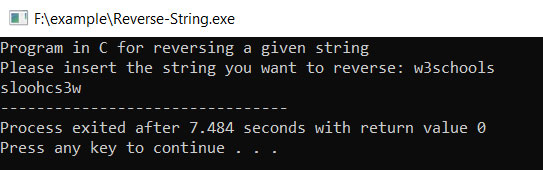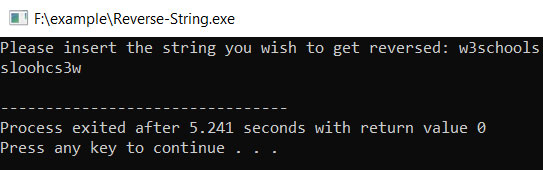## C Programming Examples Tutorial Index

String reversing is an essential concept in string related programming. There are various approaches which use different logic to reverse a string. In this chapter, you will know how to implement two different concepts and logic of reversing a string manually without using any specific predefined function.

## What is Reversing a String?

Reversing a string means the string that will be given by the user to your program in a specific sequence will get entirely reversed when the reverse of a string algorithm gets implemented in that particular input string. In the below-mentioned example, two approaches have been used to reverse a string in C language.

### Reverse the Given String by Printing It Backward

Example:

``````#include<stdio.h>
#include<string.h>

int main(void)
{
char mystrg;
int leng, g;

// Printing the program name and what the program will do
printf("Program in C for reversing a given string \n ");
printf("Please insert the string you want to reverse: ");

// fetch the input string from the user
scanf( "%s", mystrg );

// This will find the length of your string with the help of strlen() function of string.h header file
leng = strlen(mystrg);

// iterate through each and every character of the string for printing it backwards or reverse direction
for(g = leng - 1; g >= 0; g--) {
printf("%c", mystrg[g]);
}
return 0;
}``````

Output:This way you can create a simple reverse of a string program in C. There is another way around.

### Reverse the Given String by Using the Concept of Swapping

Example:

``````#include<stdio.h>

void revAString(char strg[])
{
int g, numb;
int tmpry = 0;

for(numb=0; strg[numb] != 0; numb++);
for(g = 0; g <numb/2; g++)
{
tmpry = strg[g];
strg[g]=strg[numb - 1 - g];
strg[numb - 1 - g] = tmpry;
}
for(g = 0; g < numb; g++)
putchar(strg[g]);
printf(" \n ");
}

int main(void)
{
char strg;
printf("Please insert the string you wish to get reversed: ");
scanf("%s", strg);
revAString(strg);
return 0;
}``````

Output:### Explanation

In the above program, the standard input/output header file is included in the program using the preprocessor directive #include. Then a user-defined function, revAString() is declared and in its definition, reversing the string using swapping logic is written. First of all, you take g (a counter variable), a variable numb of type integer and a temporary variable (name tmpry) used for swapping and initialize this temporary variable with 0.

Next, a for loop is implemented: for(numb=0; strg[numb] != 0; numb++); without a body which checks whether the strg[] is empty or not. If not, in the next for loop it, iteration will be done from g=0 to g less than half of the value of numb. Inside the block of for-loop, the swapping will be taking place:

``````tmpry = strg[g];

strg[g] = strg[numb - 1 - g];

strg[numb - 1 - g] = tmpry;``````

It is to be noted that for reversing a string of length n, you only need n/2 iterations. Now, once the swapping of strings is done, you need another looping to display the reversed string, which is done using this in our program:

``````for(g = 0; g < numb; g++)

putchar(strg[g]);``````

So, now the user-defined function body is ready with logic. You have to call the function from within the main(). Within the scope of main(), you have mentioned what your program does using printf().

``printf("Please insert the string you wish to get reversed: ");``

Then, using scanf(), you have taken input from the user and called the revAString() function.